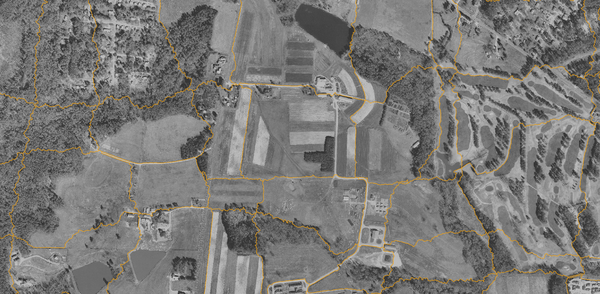## NAME

i.cutlines - Creates semantically meaningful tile borders

imagery, tiling

## SYNOPSIS

i.cutlines
i.cutlines --help
i.cutlines input=name output=name number_lines=integer edge_detection=string [existing_cutlines=name[,name,...]] no_edge_friction=integer lane_border_multiplier=integer [min_tile_size=integer] [zc_threshold=float] [zc_width=integer] [canny_low_threshold=float] [canny_high_threshold=float] [canny_sigma=float] [tile_width=integer] [tile_height=integer] [overlap=integer] processes=integer memory=integer [--overwrite] [--help] [--verbose] [--quiet] [--ui]

### Flags:

--overwrite
Allow output files to overwrite existing files
--help
Print usage summary
--verbose
Verbose module output
--quiet
Quiet module output
--ui
Force launching GUI dialog

### Parameters:

input=name [required]
Raster map to use as input for tiling
output=name [required]
Name of output vector map with cutline polygons
number_lines=integer [required]
Number of tile border lines in each direction
edge_detection=string [required]
Edge detection algorithm to use
Options: zc, canny
Default: zc
existing_cutlines=name[,name,...]
Input vector maps with existing cutlines
Or data source(s) for direct OGR access
no_edge_friction=integer [required]
Default: 5
lane_border_multiplier=integer [required]
Multiplier for borders of lanes compared to non-edge pixels
Default: 10
min_tile_size=integer
Minimum size of tiles in map units
zc_threshold=float
Sensitivity of Gaussian filter (i.zc)
Default: 1
zc_width=integer
x-y extent of the Gaussian filter (i.zc)
Default: 9
canny_low_threshold=float
Low treshold for edges (i.edge)
Default: 3
canny_high_threshold=float
High treshold for edges (i.edge)
Default: 10
canny_sigma=float
Default: 2
tile_width=integer
Width of tiles for tiled edge detection (pixels)
tile_height=integer
Height of tiles for tiled edge detection (pixels)
overlap=integer
Overlap between tiles for tiled edge detection (pixels)
Default: 1
processes=integer [required]
Number of parallel processes
Default: 1
memory=integer [required]
RAM memory available (in MB)
Default: 300

## DESCRIPTION

i.cutlines tiles the images into tiles with irregular borders that avoid cutting through meaningful objects. This allows tiling an image for parallel processing while avoiding border effects.

The approach used in i.cutlines is inspired by Soares et al (2016). The module first uses an edge detection algorithm (which the user can chose with the edge_detection parameter) to identify edges in the image. It then uses r.cost and r.drain to draw lines through the image, following edges when possible and going straight when there are none.

The user can determine the number of lines desired (number_lines) in each direction and the friction associated with pixels which are not on an edge detected in the image (no_edge_friction). The higher this value, the more the module will follow the detected edges.

In order to avoid that all lines gather into one single lowest cost path, the module defines a lane for each desired line. The parameter lane_border_multiplier defines the a multiplier of the no_edge_friction value, in order to define the cost to cross that line, i.e. the lower the value, the more likely cutlines will join each other across lanes. Output is in the form of vector polygon tiles. The user can decide a minimum size defined in map units (min_tile_size). Tiles smaller than that size will be merged with the neighboring tile they share the longest border with.

The user can provide a series of auxiliary vector maps which contain existing cutlines (roads, boundaries, etc) that the module should take into account (existing_cutlines). These can be either lines or polygons. The module will transform all to potential cutlines.

For edge detection the user can chose between the i.zc module or the i.edge addon. For the former, the user can determine the zc_threshold and the zc_width parameters. For the latter, the canny_low_threshold, canny_high_threshold and canny_sigma parameters. See the manual page of the respective modules for details about these parameters which might need tuning to fit to the specific image. As these modules read the entire image into RAM, i.cutlines allows the user to run split the image into rectangular tiles and to process each tile separately. Tiles can be defined with the tile_width, tile_height and overlap parameters. If processes is higher than one, these tiles will be processed in parallel as will the r.cost calls for the two directions.

The memory parameter determines the memory used both for the r.cost runs.

## NOTES

There is currently a parameter name conflict between i.zc and the GridModule class in pygrass. Until this is resolved tiled edge detection is only possible with i.edge.

## EXAMPLE

Using default i.zc edge detection without tiling, default parameters and 10 horizontal and 10 vertical cutlines, creating vector polygon output:
```
g.region rast=ortho_2001_t792_1m -p
i.cutlines ortho_2001_t792_1m number_lines=10 output=ortho_tiles
```Irregular vector tiles created for the NC demo data set orthophoto

## REFERENCES

Soares, Anderson Reis, Thales Sehn Körting, and Leila Maria Garcia Fonseca. 2016. "Improvements of the Divide and Segment Method for Parallel Image Segmentation." Revista Brasileira de Cartografia 68 (6), http://www.lsie.unb.br/rbc/index.php/rbc/article/view/1602.